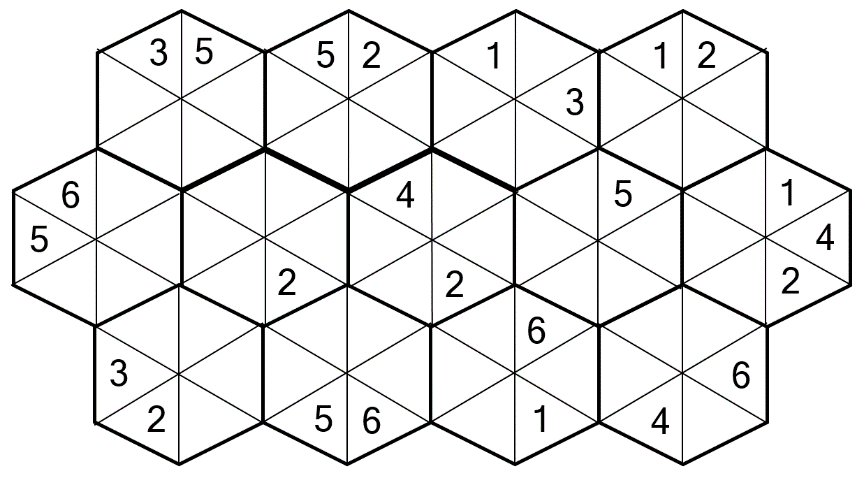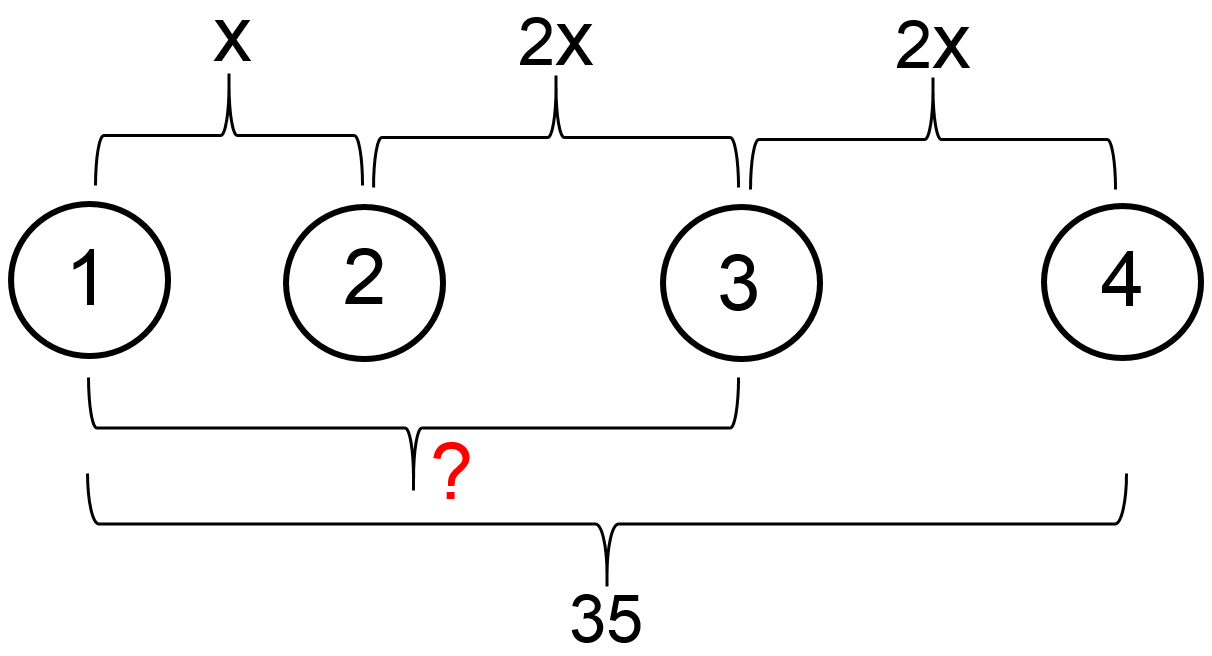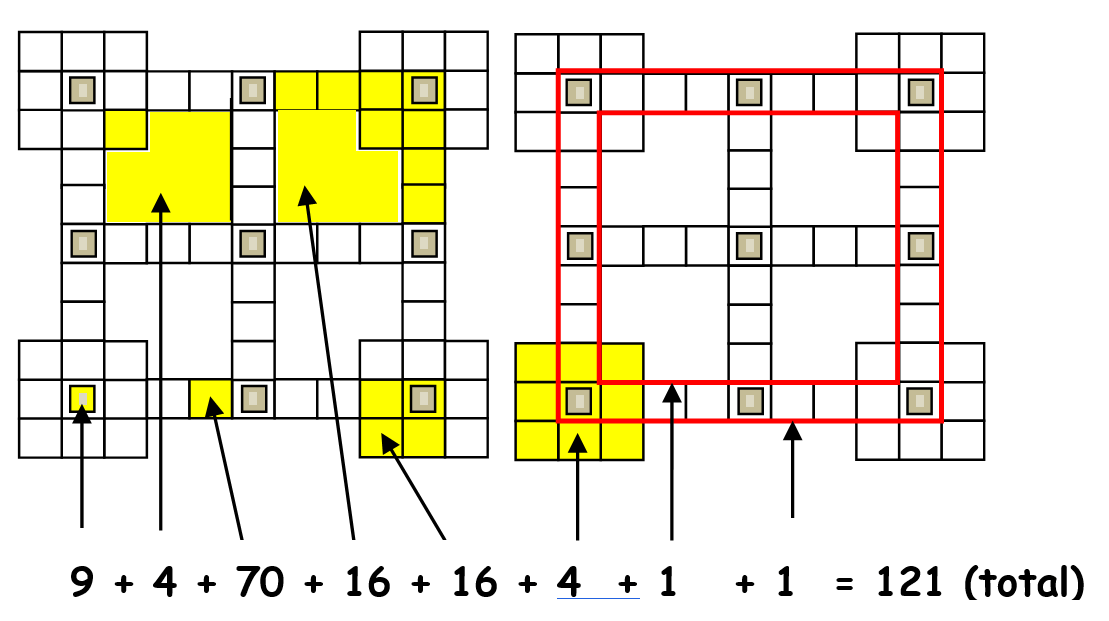Select Page

Each month, a new set of puzzles will be posted.  Come back next month for the solutions and a new set of puzzles, or subscribe to have them sent directly to you.

## MIND-Xpander Maths Problems

1. The second of five consecutive multiples of 11 is removed. The other four are then added together to give 715.  What is the lowest multiple of the remaining four? There are two possible solutions.
2. Simplify the following expression:  √ 6 x  √ 15 x  √ 10

## PRIME Numbers Puzzle

Throwing 3 darts at the following dartboard and all 3 throws must hit a number on the dartboard, what are your chances that their total will equal a Prime Number? Numbers can be used more than once.## HEXAGON-numeric puzzle (Level 1)

Fit the numbers 1 – 6 in each hexagon and where the hexagon segments touch each other, the numbers in these segments will be the same. No number can be repeated in a hexagon. Find the remaining missing numbers to complete the following puzzle.## Feedback

There are more than one way of doing these puzzles and may well be more than one answer.  Please let me and others know what alternatives you find by commenting below.  We also welcome general comments on the subject and any feedback you'd like to give.

If you have a question that needs a response from me or you would like to contact me privately, please use the contact form.

## Get more puzzles!

If you've enjoyed doing the puzzles, consider ordering the books;

• Book One - 150+ of the best puzzles
• Book Two - 200+ with new originals and more of your favourites

Both in a handy pocket sized format.   Click here for full details.

## MIND-Xpander Logic Problem

Four holes are drilled in a straight line in a rectangular steel plate.  The distance between hole 1 and 4 is 35 mm.  The distance between hole 2 and hole 3 is twice the distance between hole 1 and hole 2. The distance between hole 3 and hole 4 is the same as the distance between hole 2 and hole 3.  What is the distance in millimetres, between hole 1 and hole 3?

### SolutionGiven: Distance between holes 1 and 4 = 35mm Let x = distance between holes 1 and 2 Then, 2x = distance between holes 2 and 3, and 3 and 4. Therefore: x + 2x + 2x = 5x = 35 and then x = 7. x + 2x = 3x = 3*7 = 21mm

## Can-U-Figure-It-Out Puzzles

How many squares, of any size, are there in the following diagram?

### Solution### EQUATE+0 Puzzle

Each row, column & diagonal is an equation and you use the numbers 1 to 9 to complete the equations.   Each number can be used only once. ‘No’ numbers have been provided to get you started. Find the remaining seven numbers that satisfies all the resulting equations. Note – multiplication (x) & division (/) are performed before addition (+) and subtraction (-).

### Solution